Courses

# RD Sharma Solutions -Ex-19.2, (Part -2), Surface Area And Volume Of Right Circular Cylinder, Class 9 Class 9 Notes | EduRev

## Class 9 : RD Sharma Solutions -Ex-19.2, (Part -2), Surface Area And Volume Of Right Circular Cylinder, Class 9 Class 9 Notes | EduRev

The document RD Sharma Solutions -Ex-19.2, (Part -2), Surface Area And Volume Of Right Circular Cylinder, Class 9 Class 9 Notes | EduRev is a part of the Class 9 Course RD Sharma Solutions for Class 9 Mathematics.
All you need of Class 9 at this link: Class 9

Q12. The curved surface area of a cylinder is 1320cm2 and its base had diameter 21 cm. Find the height and volume of the cylinder.

Solution:

Let, r be the radius of the cylinder

h be the height of the cylinder

⇒ 2r = 21cm

⇒ r  = 21/2

= 10.5cm

Given, Curved surface area(CSA) = 1320cm2

⇒ 2Πrh = 1320

⇒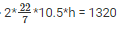⇒ h = 1320/66

⇒ h = 20 cm

Volume of cylinder =  πr2*h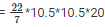= 22 * 1.5 * 10.5 * 20

= 6930cm2

Q13. The ratio between the radius of the base and the height of a cylinder is 2:3.Find the total surface area of the cylinder, if its volume is 1617cm2.

Solution:

Let, r be the radius of the cylinder

h be the height of the cylinder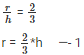Volume of cylinder =  πr2*h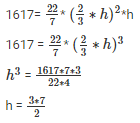h = 10.5 cm

from, eq 1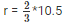= 7 cm

Total surface area of cylinder = 2πr(h+r)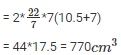Q14. A rectangular sheet of paper, 44 cm*20 cm, is rolled along its length of form cylinder. Find the volume of the cylinder so formed.

Solution:

Given, the dimensions of the sheet are 44cm*20cm

Here, length = 44 cm

Height = 20 cm

2πr = 44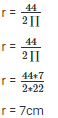Volume of cylinder = r2*h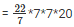= 154*20 = 3080cm3

Q15. The curved surface area of cylindrical pillar is 264m2 and its volume is 924m3. Find the diameter and the height of the pillar.

Solution:

Let, r be the radius of the cylindrical pillar

h be the height of the cylindrical pillar

CSA = 264m2

2πrh = 264m2 ——– 1

⇒ Volume of the cylinder = 924m2

Π*r2*h = 924

Πrh(r) = 924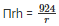Substitute πrh in eq 1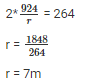substitute r value in eq 1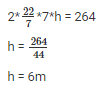so, the diameter = 2r = 2(7) = 14 m and height = 6 m

Q16. Two circular cylinders of equal volumes have their heights in the ratio 1:2. Find the ratio of two radii.

Solution:

Let, r1,r2 be the radii of the cylinder

h1,h2 be the height of the cylinder

v1,v2 be the volume of the cylinder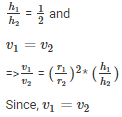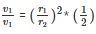⇒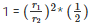⇒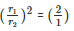⇒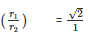Hence, the ratio of the radii are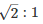Q17. The height of a right circular cylinder is 10.5 m. Three times the sum of the areas of its two circular faces is twice the area of the curved surface. Find the volume of the cylinder.

Solution:

Let, r be the radius of the right circular cylinder

h be the height of the right circular cylinder

h = 10.5 cm

⇒ 3(2πr2) = 2(2πrh)

⇒ 3r = 2h

⇒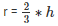⇒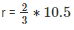⇒ r = 7cm

Volume of the cylinder = r2*h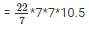= 154*10.5

= 1617cm3

Q18. How many cubic meters of earth must be dug out to sink a well 21m deep and 6 m diameter? Find the cost of plastering the inner surface as well at Rs.9.50 perm2.

Solution:

h be the height

here, h = 21m

2r = 6

⇒ r = 6/2

= 3 m

Volume of the cylinder = r2*h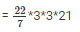= 66*9

= 594cm3

Cost of plastering = 9.5 per m3

Cost of plastering inner surface = Rs.(594*9.50) = Rs. 5643

Q19. The trunk of a tree is cylindrical and its circumference is 176 cm. If the length of the tree is 3 m. Find the volume of the timber that can be obtained from the trunk.

Solution:

We know that, circumference = 2πr

⇒176 = 2πr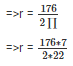⇒ r = 28 cm

Here, height(h) = 3m = 300cm

Volume of timber = r2*h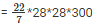= 44*8400 = 739200cm3 (or) 0.7392m3

Q20. A well with 14 m diameter is dug 8 m deep. The earth taken out of it has been evenly spread all around it to a width of 21 m to form an embankment. Find the height of the embankment.

Solution:

Let, r be the radius of well

h be the height of well

here, h = 8m

2r = 14

⇒ r = 14/2

= 7m

Volume of well = r2*h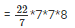= 22*56

= 1232m3

Let, re be the radius of embankment

hbe the height of embankment

Volume of well  = Volume of embankmentQ21. The difference between inside and outside surfaces of a cylindrical tube is 14 cm long is 88 sq.cm. If the volume of the tube is 176 cubic cm, Find the inner and outer radii of the tube.

Solution:

Let, R be the outer radius

Here, h = 14cm

2πRh – 2πrh = 88

⇒ 2πh(R – r) = 88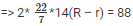⇒ (R – r) = 1cm    ——— 1

Volume of tube = π*R2*h – π*r2*h

176 = πh(R2 – r2)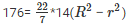⇒ (R2 – r2) = 4

⇒ (R + r)(R – r)  = 4

Here, (R –  r) = 1

⇒ (R + r)(1) = 4

⇒ (R + r) = 4 cm

⇒ R = 4 – r         ———— 2

Here , R – r = 1

⇒ R = 1 + r

Substitute R value in eq 2

⇒ 1 +  r = 4 – r

⇒  2r = 3

⇒ r = 32

= 1.5 cm

Substitute ‘r’ value in eq 1

⇒ R – 1.5 = 1

⇒ R = 1 + 1.5

⇒ R = 2.5 cm

Hence, the value of inner radii is 1.5 cm and radius of outer radii is 2.5 cm

Q.22. Water flows out through a circular pipe whose internal diameter is 2 cm, at the rate of 6 meters per second into a cylindrical tank. The water is collected in a cylindrical vessel radius of whose base is 60 cm. Find the rise in the level of water in 30 minutes?

Solution:

Given data is as follows :

Internal diameter of the pipe = 2 cm

Water flow rate through the pipe =  6 m/sec

Radius of the tank = 60 cm

Time = 30 minutes

The volume of water that flows for 1 sec through the pipe at the rate of 6 m/sec is nothing but the volume of the cylinder with n = 6

Also, given is the diameter which is 2 cm. Therefore ,

R = 1 cm

Since the speed with which water flows through the pipe is in meters/second, let us convert the radius of the pipe from centimeters to meters . Therefore ,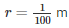Volume of water collected in the tank after  30 minutes  = Volume of water that flows through the pipe for 30 minutes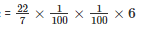Now , we have to find the volume of water that flows for 30 minutes .

Since , speed of water is in metres/second , let us convert 30 minutes into seconds . It will be 30×60

Volume of water that flows for 30 minutes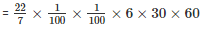Now , considering the tank , we have been given the radius of tank in centimeters . Let us first convert it into metres . Let radius of tank be ‘R’ .

R = 60 cm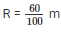Volume of water collected in the tank after  30 minutes  = Volume of water that flows through the pipe for 30 minutes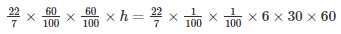h = 3 m

Therefore , the height of the tank is 3 metres

Offer running on EduRev: Apply code STAYHOME200 to get INR 200 off on our premium plan EduRev Infinity!

91 docs

,

,

,

,

,

,

,

,

,

,

,

,

,

,

,

,

,

,

,

,

,

,

,

,

,

,

,

,

,

,

;